Apertium has moved from SourceForge to GitHub.
If you have any questions, please come and talk to us on #apertium on irc.freenode.net or contact the GitHub migration team.

# Unigram tagger

Jump to: navigation, search

apertium-tagger from “m5w/apertium” supports all the unigram models from “A set of open-source tools for Turkish natural language processing.”

## Installation

First, install all prerequisites. See “If you want to add language data / do more advanced stuff.”

Then, replace <directory> with the directory you’d like to clone “m5w/apertium” into and clone the repository.

git clone https://github.com/m5w/apertium.git <directory>


Then, configure your environment and finally configure, build, and install “m5w/apertium.”

## Usage

See apertium-tagger --help .

### Training a Model on a Hand-Tagged Corpus

First, get a hand-tagged corpus as you would for any non-unigram model.

$cat handtagged.txt ^a/a<a>$
^a/a<b>$^a/a<b>$
^aa/a<a>+a<a>$^aa/a<a>+a<b>$
^aa/a<a>+a<b>$^aa/a<b>+a<a>$
^aa/a<b>+a<a>$^aa/a<b>+a<a>$
^aa/a<b>+a<b>$^aa/a<b>+a<b>$
^aa/a<b>+a<b>$^aa/a<b>+a<b>$


Example 2.1.1: handtagged.txt : a Hand-Tagged Corpus for apertium-tagger

Then, replace MODEL with the unigram model from “A set of open-source tools for Turkish natural language processing” you’d like to use, replace SERIALISED_BASIC_TAGGER with the filename to which you’d like to write the model, and train the tagger.

$apertium-tagger -s 0 -u MODEL SERIALISED_BASIC_TAGGER handtagged.txt  ### Disambiguation Either write your input to a file or pipe it to the tagger. $ cat raw.txt
^a/a<a>/a<b>/a<c>$^aa/a<a>+a<a>/a<a>+a<b>/a<b>+a<a>/a<b>+a<b>/a<a>+a<c>/a<c>+a<a>/a<c>+a<c>$


Example 2.2.1: raw.txt : Input for apertium-tagger

Replace MODEL with the unigram model from “A set of open-source tools for Turkish natural language processing” you’d like to use, replace SERIALISED_BASIC_TAGGER with the file to which you wrote the unigram model, and disambiguate the input.

$apertium-tagger -gu MODEL SERIALISED_BASIC_TAGGER raw.txt ^a/a<b>$
^aa/a<b>+a<b> echo '^a/a<a>/a<b>/a<c>$^aa/a<a>+a<a>/a<a>+a<b>/a<b>+a<a>/a<b>+a<b>/a<a>+a<c>/a<c>+a<a>/a<c>+a<c>$' | \
apertium-tagger -gu MODEL SERIALISED_BASIC_TAGGER
^a/a<b>$^aa/a<b>+a<b>$


## Unigram Models

See section 5.3 of “A set of open-source tools for Turkish natural language processing.”

### Model 1

See section 5.3.1 of “A set of open-source tools for Turkish natural language processing.”

This model assigns each analysis string a score of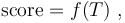\begin{align} \mathrm{score} &= f(T)~\text{,} \end{align}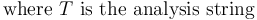\begin{align} \text{where}~&T~\text{is the analysis string} \end{align}

with additive smoothing.

Consider the following corpus.

$cat handtagged.txt ^a/a<a>$
^a/a<b>$^a/a<b>$


Example 3.1.1: handtagged.txt : A Hand-Tagged Corpus for apertium-tagger

Given the lexical unit ^a/a<a>/a<b>/a<c> , the tagger assigns the analysis string a<a> a score of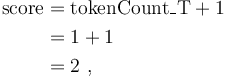\begin{align} \mathrm{score} &= \mathrm{tokenCount\_T} + 1\\ &= 1 + 1\\ &= 2~\text{,} \end{align}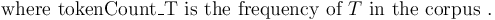\begin{align} \text{where}~&\mathrm{tokenCount\_T}~\text{is the frequency of}~T~\text{in the corpus}~\text{.} \end{align} The tagger then assigns the analysis string a<b> a score of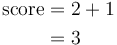\begin{align} \mathrm{score} &= 2 + 1\\ &= 3 \end{align} and the unknown analysis string a<c> a score of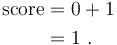\begin{align} \mathrm{score} &= 0 + 1\\ &= 1~\text{.} \end{align} If ./autogen.sh is passed the option --enable-debug , the tagger prints such calculations to standard error.  ./autogen.sh --enable-debug
$make$ echo '^a/a<a>/a<b>/a<c>$' | apertium-tagger -gu 1 SERIALISED_BASIC_TAGGER score("a<a>") == 2 == 2.000000000000000000 score("a<b>") == 3 == 3.000000000000000000 score("a<c>") == 1 == 1.000000000000000000 ^a<b>$


#### Training on Corpora with Ambiguous Lexical Units

Consider the following corpus.

$cat handtagged.txt ^a/a<a>$
^a/a<a>/a<b>$^a/a<b>$
^a/a<b>$ Example 3.1.1.1: handtagged.txt : a Hand-Tagged Corpus for apertium-tagger The tagger expects lexical units of 1 analysis string, or lexical units of size 1. However, the size of the lexical unit ^a/a<a>/a<b>$ is 2. For this lexical unit,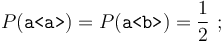$P(\texttt{a}) = P(\texttt{a}) = \frac12~\text{;}$

the tagger must effectively increment the frequency of both analysis strings by 0.500000000000000000 . However, the tagger can’t increment the analysis strings’ frequencies by a non-integral number because model 1 represents analysis strings’ frequencies as std::size_t .

Instead, the tagger multiplies all the stored analysis strings’ frequencies by this lexical unit’s size and increments the frequency of each of this lexical unit’s analysis strings by 1.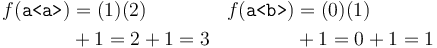\begin{align} f(\texttt{a}) &= (1)(2) &f(\texttt{a}) &= (0)(1)\\ &+ 1 = 2 + 1 = 3 & &+ 1 = 0 + 1 = 1 \end{align}

The tagger could then increment the analysis strings’ frequencies of another lexical unit of size 2 without multiplying any of the stored analysis strings’ frequencies. To account for this, the tagger stores the least common multiple of all lexical units’ sizes; only if the LCM isn’t divisible by a lexical unit’s size does the tagger multiply all the analysis strings’ frequencies.

After incrementing the analysis strings’ frequencies of the lexical unit ^a/a<a>/a<b>$, the tagger increments the analysis string a<b> of the lexical unit ^a/a<b>$ by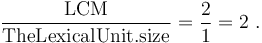\begin{align} \frac{\mathrm{LCM}}{\mathrm{TheLexicalUnit.size}} = \frac{2}{1} = 2~\text{.} \end{align}

If the tagger gets another lexical unit of size 2, it would increment the frequency of each of the lexical unit’s analysis strings by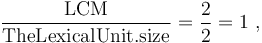\begin{align} \frac{\mathrm{LCM}}{\mathrm{TheLexicalUnit.size}} = \frac{2}{2} = 1~\text{,} \end{align}

and if it gets a lexical unit of size 3, it would multiply all the analysis strings’ frequencies by 3 and then increment the frequency of each of the lexical unit’s analysis strings by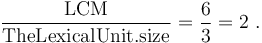\begin{align} \frac{\mathrm{LCM}}{\mathrm{TheLexicalUnit.size}} = \frac{6}{3} = 2~\text{.} \end{align}

Each model supports functions to increment all their stored analysis strings’ frequencies, so models 2 and 3 support this algorithm as well.

TODO: If one passes the -d option to apertium-tagger , the tagger prints warnings about ambiguous analyses in corpora to stderr.

$apertium-tagger -ds 0 -u 1 handtagged.txt apertium-tagger: handtagged.txt: 2:13: unexpected analysis "a<b>" following anal ysis "a<a>" ^a/a<a>/a<b>$
^


#### File Format

The tagger represents this model as std::map<Analysis, std::size_t> Model; .

It first serialises Model.size() , the size of the parameter vector of analysis strings, which is of type std::size_t , followed by analysis string-frequency pairs.

To reduce file size, the it writes only the non-zero bytes of a std::size_t  preceded by the number of bytes to read.

[. . . .]

([. . .]).serialise(0x00000000, [. . .]); // 00
([. . .]).serialise(0x000000ff, [. . .]); // 01ff
([. . .]).serialise(0x0000ffff, [. . .]); // 02ffff
([. . .]).serialise(0x00ffffff, [. . .]); // 03ffffff
([. . .]).serialise(0xffffffff, [. . .]); // 04ffffffff

[. . . .]


Example 3.2.1: std::size_t  Serialisation

The tagger serialises the analysis string-frequency pairs, which are of type std::pair<Analysis, std::size_t> . For each analysis string-frequency pair, it first serialises the analysis string, followed by the frequency, which is of type std::size_t . It serialises TheMorphemes , the sequence of morphemes, which is of type std::vector<Morpheme> . The tagger first serialises TheMorphemes.size() , the size of the sequence of morphemes, which is of type std::size_t , followed by the morphemes. For each morpheme, it first serialises the lemma, followed by the sequence of tags. The tagger serialises TheLemma , the lemma, which is of type std::wstring . It first serialises TheLemma.size() , the length of the lemma, which is of type std::size_t , followed by the lemma itself.

The tagger then serialises TheTags.size() , the size of the sequence of tags, which is of type std::size_t , followed by the tag sequence. For each tag, it first serialises TheTag.size() , the length of the tag, followed by the tag itself.

Given the corpus

^a/a<b>$ the tagger writes  0000000: 0101 0101 0101 0161 0101 0101 0161 0101 .......a.....b.. 0000010: 0a .  ### Model 2 See section 5.3.2 of “A set of open-source tools for Turkish natural language processing.” Consider Example 3.1.1: handtagged.txt . The tag string <b> is twice as frequent as <a>. However, model 1 scores b<a> and b<b> equally because neither analysis string appears in the corpus. This model splits each analysis string into a root, r , and the part of the analysis string that isn’t the root, a . An analysis string’s root is its first lemma. The r of a<b>+c<d> is a , and its a is <b>+c<d> . The tagger assigns each analysis string a score of P(r | a)f(a) with add-one smoothing. (Without additive smoothing, this model would be the same as model 1.) The tagger assigns higher scores to unknown analysis strings with frequent a than to unknown analysis strings with infrequent a . Given the lexical unit ^b/b<a>/b<b>$, the tagger assigns the analysis string b<a> a score of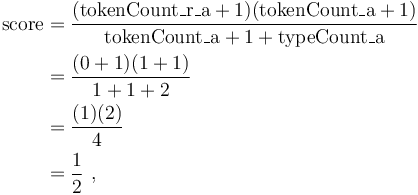\begin{align} \mathrm{score} & = \frac{(\mathrm{tokenCount\_r\_a} + 1)(\mathrm{tokenCount\_a} + 1)}{\mathrm{tokenCount\_a} + 1 + \mathrm{typeCount\_a}} \\ & = \frac{(0 + 1)(1 + 1)}{1 + 1 + 2} \\ & = \frac{(1)(2)}{4} \\ & = \frac{1}{2}~\text{,} \end{align}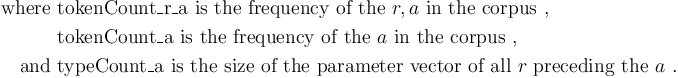\begin{align} \text{where}~&\mathrm{tokenCount\_r\_a}~\text{is the frequency of the}~r,a~\text{in the corpus ,} \\ &\mathrm{tokenCount\_a}~\text{is the frequency of the}~a~\text{in the corpus ,} \\ \text{and}~&\mathrm{typeCount\_a}~\text{is the size of the parameter vector of all}~r~\text{preceding the}~a~\text{.} \end{align}

Note that typeCount_a counts the analysis string being scored. For example, the tagger would assign the known analysis string a<a> a score of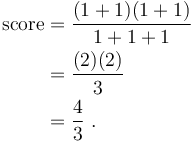\begin{align} \mathrm{score} & = \frac{(1 + 1)(1 + 1)}{1 + 1 + 1} \\ & = \frac{(2)(2)}{3} \\ & = \frac{4}{3}~\text{.} \end{align}

The tagger assigns the analysis string b<b> a score of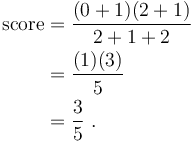\begin{align} \mathrm{score} & = \frac{(0 + 1)(2 + 1)}{2 + 1 + 2} \\ & = \frac{(1)(3)}{5} \\ & = \frac{3}{5}~\text{.} \end{align}

#### File Format

The tagger represents this model as std::map<a, std::map<Lemma, std::size_t> > Model; .

See section 3.1.2.

For each a , the tagger first serialises TheTags , the tag sequence, which is of type std::vector<Tag> , followed by TheMorphemes , the morpheme sequence, which is of type std::vector<Morpheme> . For each Lemma , the tagger serialises TheLemma , the lemma, which is of type std::wstring .

Given the corpus

^a/a<b>+c<d>$ the tagger writes  0000000: 0101 0101 0101 0162 0101 0101 0163 0101 .......b.....c.. 0000010: 0101 0164 0101 0101 0161 0101 0a ...d.....a...  ### Model 3 See section 5.3.3 of “A set of open-source tools for Turkish natural language processing.” Consider Example 3.1.1: handtagged.txt . The morpheme a<b> is twice as frequent as the morpheme a<a> . However, model 2 scores the analysis strings a<a>+a<a> and a<b>+a<a> equally because the a of neither appears in the corpus. This model splits each analysis string into an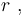$r~\text{,}$ a first inflection,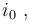$i_0~\text{,}$ and a sequence of derivation-inflection pairs,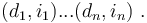$(d_1,i_1)...(d_n,i_n)~\text{.}$ The r of the analysis string a<b>+c<d> is a , its i0 is <b> , and its (d1,i1)...(dn,in) is c<d> , where its d1 is c , and its i1 is <d> . The tagger assigns each analysis string a score of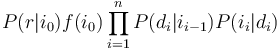$P(r|i_0)f(i_0)\prod_{i = 1}^n P(d_i|i_{i-1})P(i_i|d_i)$ with add-one smoothing. The tagger assigns higher scores to unknown analysis strings with frequent r,i0 than to unknown analysis strings with infrequent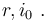$r,i_0~\text{.}$ Given the lexical unit ^aa/a<a>+a<a>/a<b>+a<a>$ , the tagger assigns the analysis string a<a>+a<a> a score of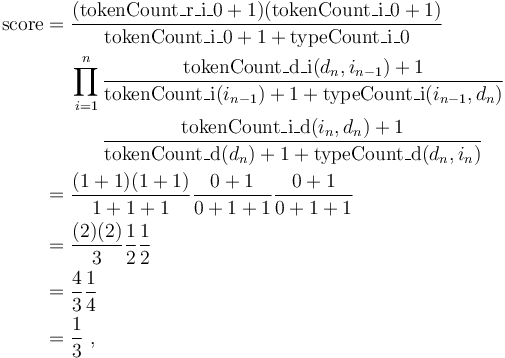\begin{align} \mathrm{score} =\;&\frac{(\mathrm{tokenCount\_r\_i\_0} + 1)(\mathrm{tokenCount\_i\_0} + 1)}{\mathrm{tokenCount\_i\_0} + 1 + \mathrm{typeCount\_i\_0}}\\ &\begin{align}\prod_{i = 1}^n\,&\frac{\mathrm{tokenCount\_d\_i}(d_n, i_{n - 1}) + 1}{\mathrm{tokenCount\_i}(i_{n - 1}) + 1 + \mathrm{typeCount\_i}(i_{n - 1}, d_n)}\\ &\frac{\mathrm{tokenCount\_i\_d}(i_n, d_n) + 1}{\mathrm{tokenCount\_d}(d_n) + 1 + \mathrm{typeCount\_d}(d_n, i_n)}\end{align}\\ =\;&\frac{(1 + 1)(1 + 1)}{1 + 1 + 1}\frac{0 + 1}{0 + 1 + 1}\frac{0 + 1}{0 + 1 + 1}\\ =\;&\frac{(2)(2)}3\frac12\frac12\\ =\;&\frac43\frac14\\ =\;&\frac13~\text{,} \end{align}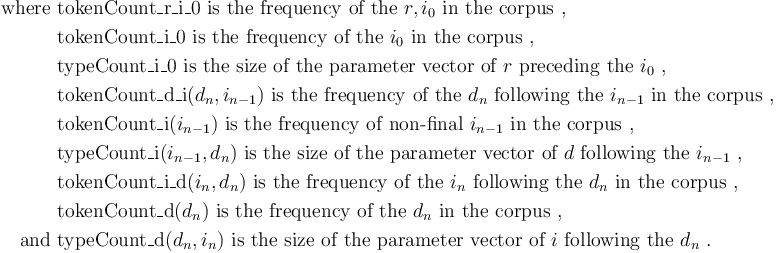\begin{align} \text{where}~&\mathrm{tokenCount\_r\_i\_0}~\text{is the frequency of the}~r,i_0~\text{in the corpus}~\text{,}\\ &\mathrm{tokenCount\_i\_0}~\text{is the frequency of the}~i_0~\text{in the corpus}~\text{,}\\ &\mathrm{typeCount\_i\_0}~\text{is the size of the parameter vector of}~r~\text{preceding the}~i_0~\text{,}\\ &\mathrm{tokenCount\_d\_i}(d_n, i_{n - 1})~\text{is the frequency of the}~d_n~\text{following the}~i_{n - 1}~\text{in the corpus}~\text{,}\\ &\mathrm{tokenCount\_i}(i_{n - 1})~\text{is the frequency of non-final}~i_{n - 1}~\text{in the corpus}~\text{,}\\ &\mathrm{typeCount\_i}(i_{n - 1}, d_n)~\text{is the size of the parameter vector of}~d~\text{following the}~i_{n - 1}~\text{,}\\ &\mathrm{tokenCount\_i\_d}(i_n, d_n)~\text{is the frequency of the}~i_n~\text{following the}~d_n~\text{in the corpus}~\text{,}\\ &\mathrm{tokenCount\_d}(d_n)~\text{is the frequency of the}~d_n~\text{in the corpus}~\text{,}\\ \text{and}~&\mathrm{typeCount\_d}(d_n, i_n)~\text{is the size of the parameter vector of}~i~\text{following the}~d_n~\text{.} \end{align}

The tagger assigns the analysis string a<b>+a<a> a score of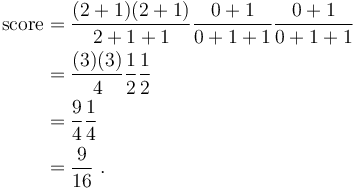\begin{align} \mathrm{score} =\;&\frac{(2 + 1)(2 + 1)}{2 + 1 + 1}\frac{0 + 1}{0 + 1 + 1}\frac{0 + 1}{0 + 1 + 1}\\ =\;&\frac{(3)(3)}{4}\frac12\frac12\\ =\;&\frac94\frac14\\ =\;&\frac9{16}~\text{.} \end{align}

#### File Format

The tagger represents this model as std::pair<std::map<i, std::map<Lemma, std::size_t> >, std::pair<std::map<i, std::map<Lemma, std::size_t> >, std::map<Lemma, std::map<i, std::size_t> > > > Model; .

See section 3.1.2.

For each i , the tagger serialises TheTags , the tag sequence, which is of type std::vector<Tag> .

Given the corpus

^a/a<b>+c<d>\$


the tagger writes 

0000000: 0101 0101 0101 0162 0101 0101 0161 0101 .......b.....a..
0000010: 0101 0101 0101 0162 0101 0101 0163 0101 .......b.....c..
0000020: 0101 0101 0163 0101 0101 0101 0164 0101 .....c.......d..
0000030: 0a                                      .